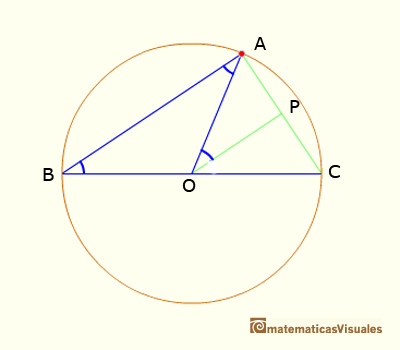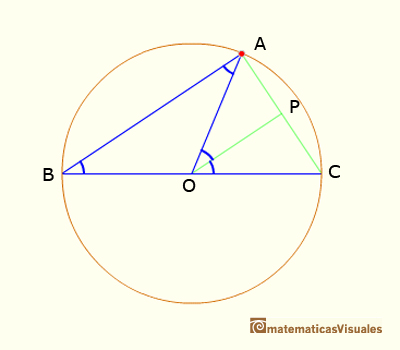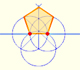Central and Inscribed Angle in a Circle: Case IICentral angle in a circle is twice the angle inscribed in the circle.

CASE 2. When the vertex is one of the ends of the diameter and the cord draws up from the other end, the angle in center is the double of the angle in the vertex.In this case we want to prove that

We draw the line OP parallel to AB, and thenAdding this two angles we get the result:

Now is very easy to probe the general case. You can see an interactive demostration of the Central and inscribed angles in a circle | General Case.

REFERENCES

Euclides, The ElementsCentral angle in a circle is twice the angle inscribed in the circle.Using a ruler and a compass we can draw fifteen degrees angles. These are basic examples of the central and inscribed in a circle angles property.Each point in the circle circunscribed to a triangle give us a line (Wallace-Simson line)Interactive demonstration of the Wallace-Simson line.You can draw a regular pentagon given one of its sides constructing the golden ratio with ruler and compass.In his book 'Underweysung der Messung' Durer draw a non-regular pentagon with ruler and a fixed compass. It is a simple construction and a very good approximation of a regular pentagon.The diagonal of a regular pentagon are in golden ratio to its sides and the point of intersection of two diagonals of a regular pentagon are said to divide each other in the golden ratio or 'in extreme and mean ratio'.